CGAL 5.1 - 3D Polyhedral Surface
CGAL::Polyhedron_3< Traits > Class Template Reference

#include <CGAL/Polyhedron_3.h>

## Definition

A polyhedral surface Polyhedron_3 consists of vertices V, edges E, facets F and an incidence relation on them.

Each edge is represented by two halfedges with opposite orientations.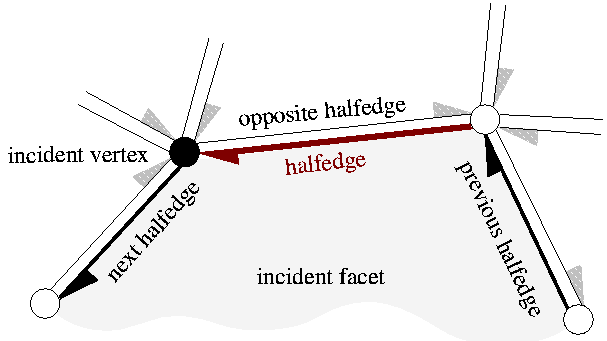Vertices represent points in 3d-space. Edges are straight line segments between two endpoints. Facets are planar polygons without holes defined by the circular sequence of halfedges along their boundary. The polyhedral surface itself can have holes. The halfedges along the boundary of a hole are called border halfedges and have no incident facet. An edge is a border edge if one of its halfedges is a border halfedge. A surface is closed if it contains no border halfedges. A closed surface is a boundary representation for polyhedra in three dimensions. The convention is that the halfedges are oriented counterclockwise around facets as seen from the outside of the polyhedron. An implication is that the halfedges are oriented clockwise around the vertices. The notion of the solid side of a facet as defined by the halfedge orientation extends to polyhedral surfaces with border edges although they do not define a closed object. If normal vectors are considered for the facets, normals point outwards (following the right hand rule).

The strict definition can be found in . One implication of this definition is that the polyhedral surface is always an orientable and oriented 2-manifold with border edges, i.e., the neighborhood of each point on the polyhedral surface is either homeomorphic to a disc or to a half disc, except for vertices where many holes and surfaces with boundary can join. Another implication is that the smallest representable surface is a triangle (for polyhedral surfaces with border edges) or a tetrahedron (for polyhedra). Boundary representations of orientable 2-manifolds are closed under Euler operations. They are extended with operations that create or close holes in the surface.

Other intersections besides the incidence relation are not allowed, although they are not automatically handled, since self intersections are not easy to check efficiently. Polyhedron_3 does only maintain the combinatorial integrity of the polyhedral surface (using Euler operations) and does not consider the coordinates of the points or any geometric information.

The class Polyhedron_3 can represent polyhedral surfaces as well as polyhedra. The interface is designed in such a way that it is easy to ignore border edges and work only with polyhedra.

The sequence of edges can be ordered in the data structure on request such that the sequence starts with the non-border edges and ends with the border edges. Border edges are then itself ordered such that the halfedge which is incident to the facet comes first and the halfedge incident to the hole comes thereafter. This normalization step counts simultaneously the number of border edges. This number is zero if and only if the surface is a closed polyhedron. Note that this class does not maintain this counter nor the halfedge order during further modifications. There is no automatic caching done for auxiliary information.

Parameters

The full template declaration of Polyhedron_3 states four template parameters:

template < class PolyhedronTraits_3,
template < class T, class I>
class Alloc = CGAL_ALLOCATOR(int) >

The first parameter requires a model of the PolyhedronTraits_3 concept as argument, for example CGAL::Polyhedron_traits_3. The second parameter expects a model of the PolyhedronItems_3 concept. By default, the class CGAL::Polyhedron_items_3 is preselected. The third parameter is a class template. A model of the HalfedgeDS concept is expected. By default, the class CGAL::HalfedgeDS_default is preselected, which is a list based implementation of the halfedge data structure. The fourth parameter Alloc requires a standard allocator for STL container classes. The rebind mechanism from Alloc will be used to create appropriate allocators internally. A default is provided with the macro CGAL_ALLOCATOR(int) from the <CGAL/memory.h> header file.

CGAL::Polyhedron_3::Vertex
CGAL::Polyhedron_3::Halfedge
CGAL::Polyhedron_3::Facet
PolyhedronTraits_3
CGAL::Polyhedron_traits_3<Kernel>
PolyhedronItems_3
CGAL::Polyhedron_items_3
HalfedgeDS
CGAL::HalfedgeDS_default
CGAL::Polyhedron_incremental_builder_3<HDS>
CGAL::Modifier_base.

Example

This example program instantiates a polyhedron using the default traits class and creates a tetrahedron.

#include <CGAL/Simple_cartesian.h>
#include <CGAL/Polyhedron_3.h>
typedef CGAL::Polyhedron_3<Kernel> Polyhedron;
typedef Polyhedron::Halfedge_handle Halfedge_handle;
int main() {
Polyhedron P;
Halfedge_handle h = P.make_tetrahedron();
if ( P.is_tetrahedron(h))
return 0;
return 1;
}
Examples:
Polyhedron/draw_polyhedron.cpp, Polyhedron/polyhedron_prog_color.cpp, Polyhedron/polyhedron_prog_cube.cpp, Polyhedron/polyhedron_prog_incr_builder.cpp, Polyhedron/polyhedron_prog_normals.cpp, Polyhedron/polyhedron_prog_off.cpp, Polyhedron/polyhedron_prog_planes.cpp, Polyhedron/polyhedron_prog_simple.cpp, Polyhedron/polyhedron_prog_subdiv.cpp, Polyhedron/polyhedron_prog_tetra.cpp, Polyhedron/polyhedron_prog_vector.cpp, and Polyhedron_IO/polyhedron2vrml.cpp.

## Classes

class  Facet
A facet optionally stores a plane equation, and a reference to an incident halfedge that points to the facet. More...

class  Halfedge
A halfedge is an oriented edge between two vertices. More...

class  Vertex
A vertex optionally stores a point and a reference to an incident halfedge that points to the vertex. More...

## Related Functions

(Note that these are not member functions.)

template<class PolyhedronTraits_3 >
bool read_off (std::istream &in, Polyhedron_3< PolyhedronTraits_3 > &P)

template<class PolyhedronTraits_3 >
std::istream & operator>> (std::istream &in, Polyhedron_3< PolyhedronTraits_3 > &P)

template<class PolyhedronTraits_3 >
bool write_off (std::ostream &out, Polyhedron_3< PolyhedronTraits_3 > &P)

template<class PolyhedronTraits_3 >
std::ostream & operator<< (std::ostream &out, Polyhedron_3< PolyhedronTraits_3 > &P)

## Types

typedef unspecified_type Items
items class selected for PolyhedronItems_3.

typedef unspecified_type HalfedgeDS
instantiated halfedge data structure.

typedef unspecified_type size_type
size type of HalfedgeDS.

typedef unspecified_type difference_type
difference type of HalfedgeDS.

typedef unspecified_type iterator_category
iterator category of HalfedgeDS for all iterators.

typedef unspecified_type circulator_category
circulator category of all circulators; bidirectional category if the Items::Halfedge provides a Halfedge::prev() member function, otherwise forward category.

typedef unspecified_type allocator_type
allocator type Alloc.

## Handles, Iterators, and Circulators

The following handles, iterators, and circulators have appropriate non-mutable counterparts, i.e., const_handle, const_iterator, and const_circulator.

The mutable types are assignable to their non-mutable counterparts. Both circulators are assignable to the Halfedge_iterator. The iterators are assignable to the respective handle types. Wherever the handles appear in function parameter lists, the corresponding iterators can be used as well. For convenience, the Edge_iterator enumerates every other halfedge. It is based on the CGAL::N_step_adaptor class. For convenience, the Point_iterator enumerates all points in the polyhedral surface in the same order as the Vertex_iterator, but with the value type Point. Similarly, a Plane_iterator is provided.

All handles are model of LessThanComparable and Hashable, that is they can be used as keys in containers such as std::map and boost::unordered_map.

typedef unspecified_type Point_3
point stored in vertices.

typedef unspecified_type Plane_3
plane equation stored in facets (if supported).

typedef unspecified_type Vertex_handle
handle to vertex.

typedef unspecified_type Halfedge_handle
handle to halfedge.

typedef unspecified_type Facet_handle
handle to facet.

typedef unspecified_type Vertex_iterator
iterator over all vertices.

typedef unspecified_type Halfedge_iterator
iterator over all halfedges.

typedef unspecified_type Facet_iterator
iterator over all facets.

typedef unspecified_type Halfedge_around_vertex_circulator
circulator of halfedges around a vertex (cw).

typedef unspecified_type Halfedge_around_facet_circulator
circulator of halfedges around a facet (ccw).

typedef unspecified_type Edge_iterator
iterator over all edges (every other halfedge).

typedef unspecified_type Point_iterator
iterator over all points.

typedef unspecified_type Plane_iterator
iterator over all plane equations.

## Types for Tagging Optional Features

The following types are equal to either Tag_true or Tag_false , depending on whether the named feature is supported or not.

typedef unspecified_type Supports_vertex_halfedge
Vertex::halfedge().

typedef unspecified_type Supports_vertex_point
Vertex::point().

typedef unspecified_type Supports_halfedge_prev
Halfedge::prev().

typedef unspecified_type Supports_halfedge_vertex
Halfedge::vertex().

typedef unspecified_type Supports_halfedge_facet
Halfedge::facet().

typedef unspecified_type Supports_facet_halfedge
Facet::halfedge().

typedef unspecified_type Supports_facet_plane
Facet::plane().

typedef unspecified_type Supports_removal
supports removal of individual elements.

## Creation

Polyhedron_3 (const Traits &traits=Traits())
Default constructor.

Polyhedron_3 (size_type v, size_type h, size_type f, const Traits &traits=Traits())
Constructor of a polyhedron with storage reserved for v vertices, h halfedges, and f facets. More...

void reserve (size_type v, size_type h, size_type f)
reserve storage for v vertices, h halfedges, and f facets. More...

Halfedge_handle make_tetrahedron ()
a tetrahedron is added to the polyhedral surface. More...

Halfedge_handle make_tetrahedron (const Point &p1, const Point &p2, const Point &p3, const Point &p4)
a tetrahedron is added to the polyhedral surface with its vertices initialized to p1, p2, p3, and p4. More...

Halfedge_handle make_triangle ()
a triangle with border edges is added to the polyhedral surface. More...

Halfedge_handle make_triangle (const Point &p1, const Point &p2, const Point &p3)
a triangle with border edges is added to the polyhedral surface with its vertices initialized to p1, p2, and p3. More...

## Access Member Functions

bool empty () const
returns true if the polyhedron is empty.

size_type size_of_vertices () const
number of vertices.

size_type size_of_halfedges () const
number of halfedges (incl. border halfedges).

size_type size_of_facets () const
number of facets.

size_type capacity_of_vertices () const
space reserved for vertices.

size_type capacity_of_halfedges () const
space reserved for halfedges.

size_type capacity_of_facets () const
space reserved for facets.

size_t bytes () const
bytes used for the polyhedron.

size_t bytes_reserved () const
bytes reserved for the polyhedron.

allocator_type get_allocator () const
allocator object.

Vertex_iterator vertices_begin ()
iterator over all vertices.

Vertex_iterator vertices_end ()
past-the-end iterator.

Halfedge_iterator halfedges_begin ()
iterator over all halfedges.

Halfedge_iterator halfedges_end ()
past-the-end iterator.

Facet_iterator facets_begin ()
iterator over all facets (excluding holes).

Facet_iterator facets_end ()
past-the-end iterator.

Edge_iterator edges_begin ()
iterator over all edges.

Edge_iterator edges_end ()
past-the-end iterator.

Point_iterator points_begin ()
iterator over all points.

Point_iterator points_end ()
past-the-end iterator.

Plane_iterator planes_begin ()
iterator over all plane equations.

Plane_iterator planes_end ()
past-the-end iterator.

const Traitstraits () const
returns the traits class.

## Combinatorial Predicates

bool is_closed () const
returns true if there are no border edges.

bool is_pure_bivalent () const
returns true if all vertices have exactly two incident edges.

bool is_pure_trivalent () const
returns true if all vertices have exactly three incident edges.

bool is_pure_triangle () const
returns true if all facets are triangles.

returns true if all facets are quadrilaterals.

bool is_triangle (Halfedge_const_handle h) const
true iff the connected component denoted by h is a triangle.

bool is_tetrahedron (Halfedge_const_handle h) const
true iff the connected component denoted by h is a tetrahedron.

## Euler Operators (Combinatorial Modifications)

The following Euler operations modify consistently the combinatorial structure of the polyhedral surface. The geometry remains unchanged.

Halfedge_handle split_facet (Halfedge_handle h, Halfedge_handle g)
splits the facet incident to h and g into two facets with a new diagonal between the two vertices denoted by h and g respectively. More...

Halfedge_handle join_facet (Halfedge_handle h)
joins the two facets incident to h. More...

Halfedge_handle split_vertex (Halfedge_handle h, Halfedge_handle g)
splits the vertex incident to h and g into two vertices, the old vertex remains and a new copy is created, and connects them with a new edge. More...

Halfedge_handle join_vertex (Halfedge_handle h)
joins the two vertices incident to h. More...

Halfedge_handle split_edge (Halfedge_handle h)
splits the halfedge h into two halfedges inserting a new vertex that is a copy of h->opposite()->vertex(). More...

Halfedge_handle flip_edge (Halfedge_handle h)
performs an edge flip. More...

Halfedge_handle create_center_vertex (Halfedge_handle h)
barycentric triangulation of h->facet(). More...

Halfedge_handle erase_center_vertex (Halfedge_handle g)
reverses create_center_vertex(). More...

## Euler Operators Modifying Genus

Halfedge_handle split_loop (Halfedge_handle h, Halfedge_handle i, Halfedge_handle j)
cuts the polyhedron into two parts along the cycle (h,i,j) (edge j runs on the backside of the three dimensional figure above). More...

Halfedge_handle join_loop (Halfedge_handle h, Halfedge_handle g)
glues the boundary of the two facets denoted by h and g together and returns h. More...

## Modifying Facets and Holes

Halfedge_handle make_hole (Halfedge_handle h)
removes the incident facet of h and changes all halfedges incident to the facet into border edges. More...

Halfedge_handle fill_hole (Halfedge_handle h)
fills a hole with a newly created facet. More...

Halfedge_handle add_vertex_and_facet_to_border (Halfedge_handle h, Halfedge_handle g)
creates a new facet within the hole incident to h and g by connecting the tip of g with the tip of h with two new halfedges and a new vertex and filling this separated part of the hole with a new facet, such that the new facet is incident to g. More...

Halfedge_handle add_facet_to_border (Halfedge_handle h, Halfedge_handle g)
creates a new facet within the hole incident to h and g by connecting the vertex denoted by g with the vertex denoted by h with a new halfedge and filling this separated part of the hole with a new facet, such that the new facet is incident to g. More...

## Erasing

void erase_facet (Halfedge_handle h)
removes the incident facet of h and changes all halfedges incident to the facet into border edges or removes them from the polyhedral surface if they were already border edges. More...

void erase_connected_component (Halfedge_handle h)
removes the vertices, halfedges, and facets that belong to the connected component of h. More...

unsigned int keep_largest_connected_components (unsigned int nb_components_to_keep)
Erases the small connected components and the isolated vertices. More...

void clear ()
removes all vertices, halfedges, and facets.

## Operations with Border Halfedges

Halfedges incident to a hole are called border halfedges.

A halfedge is a border edge if itself or its opposite halfedge are border halfedges. The only requirement to work with border halfedges is that the Halfedge class provides a member function Halfedge::is_border() returning a bool. Usually, the halfedge data structure supports facets and a NULL facet pointer will indicate a border halfedge, but this is not the only possibility. The is_border() predicate divides the edges into two classes, the border edges and the non-border edges. The following normalization reorganizes the sequential storage of the edges such that the non-border edges precede the border edges, and that for each border edge the latter one of the two halfedges is a border halfedge (the first one is a non-border halfedge in conformance with the polyhedral surface definition). The normalization stores the number of border halfedges and the halfedge iterator the border edges start at within the data structure. Halfedge insertion or removal and changing the border status of a halfedge invalidate these values. They are not automatically updated.

void normalize_border ()
sorts halfedges such that the non-border edges precede the border edges. More...

size_type size_of_border_halfedges () const
number of border halfedges. More...

size_type size_of_border_edges () const
number of border edges. More...

Halfedge_iterator border_halfedges_begin ()
halfedge iterator starting with the border edges. More...

Edge_iterator border_edges_begin ()
edge iterator starting with the border edges. More...

## Miscellaneous

void inside_out ()
reverses facet orientations (incl. plane equations if supported).

bool is_valid (bool verbose=false, int level=0) const
returns true if the polyhedral surface is combinatorially consistent. More...

bool normalized_border_is_valid (bool verbose=false) const
returns true if the border halfedges are in normalized representation, which is when enumerating all halfedges with the iterator: The non-border edges precede the border edges and for border edges, the second halfedge is the border halfedge. More...

void delegate (CGAL::Modifier_base< HDS > &m)
This is an advanced function. More...

## ◆ Polyhedron_3()

template<typename Traits >
 CGAL::Polyhedron_3< Traits >::Polyhedron_3 ( size_type v, size_type h, size_type f, const Traits & traits = Traits() )

Constructor of a polyhedron with storage reserved for v vertices, h halfedges, and f facets.

The reservation sizes are a hint for optimizing storage allocation.

## Member Function Documentation

template<typename Traits >
 Halfedge_handle CGAL::Polyhedron_3< Traits >::add_facet_to_border ( Halfedge_handle h, Halfedge_handle g )

creates a new facet within the hole incident to h and g by connecting the vertex denoted by g with the vertex denoted by h with a new halfedge and filling this separated part of the hole with a new facet, such that the new facet is incident to g.

Returns the halfedge of the new edge that is incident to the new facet.

Precondition
h->is_border(), g->is_border(), h != g, h->next() != g, and g can be reached along the same hole starting with h.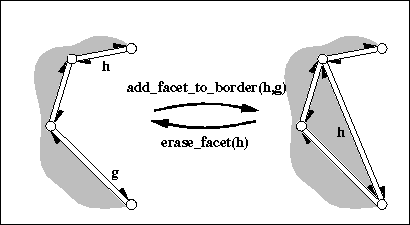template<typename Traits >
 Halfedge_handle CGAL::Polyhedron_3< Traits >::add_vertex_and_facet_to_border ( Halfedge_handle h, Halfedge_handle g )

creates a new facet within the hole incident to h and g by connecting the tip of g with the tip of h with two new halfedges and a new vertex and filling this separated part of the hole with a new facet, such that the new facet is incident to g.

Returns the halfedge of the new edge that is incident to the new facet and the new vertex.

Precondition
h->is_border(), g->is_border(), h != g, and g can be reached along the same hole starting with h.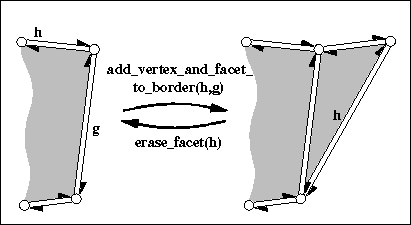## ◆ border_edges_begin()

template<typename Traits >
 Edge_iterator CGAL::Polyhedron_3< Traits >::border_edges_begin ( )

edge iterator starting with the border edges.

The range [edges_begin(), border_edges_begin()) denotes all non-border edges. The range [border_edges_begin(), edges_end()) denotes all border edges.

Precondition
last normalize_border() call still valid, see above.

## ◆ border_halfedges_begin()

template<typename Traits >
 Halfedge_iterator CGAL::Polyhedron_3< Traits >::border_halfedges_begin ( )

halfedge iterator starting with the border edges.

The range [halfedges_begin(), border_halfedges_begin()) denotes all non-border halfedges. The range [border_halfedges_begin(), halfedges_end()) denotes all border edges.

Precondition
last normalize_border() call still valid, see above.

## ◆ create_center_vertex()

template<typename Traits >
 Halfedge_handle CGAL::Polyhedron_3< Traits >::create_center_vertex ( Halfedge_handle h )

barycentric triangulation of h->facet().

Creates a new vertex, a copy of h->vertex(), and connects it to each vertex incident to h->facet() splitting h->facet() into triangles. h remains incident to the original facet, all other triangles are copies of this facet. Returns the halfedge h->next() after the operation, i.e., a halfedge pointing to the new vertex. The time is proportional to the size of the facet.

Precondition
h is not a border halfedge.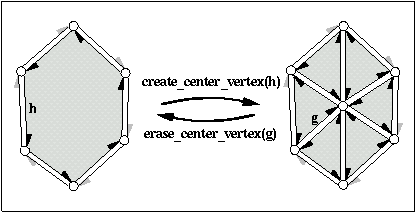## ◆ delegate()

template<typename Traits >
 void CGAL::Polyhedron_3< Traits >::delegate ( CGAL::Modifier_base< HDS > & m )

calls the Modifier_base::operator()() of the modifier m.

Precondition
The polyhedral surface must be valid when the modifier returns from execution.

## ◆ erase_center_vertex()

template<typename Traits >
 Halfedge_handle CGAL::Polyhedron_3< Traits >::erase_center_vertex ( Halfedge_handle g )

reverses create_center_vertex().

Erases the vertex pointed to by g and all incident halfedges thereby merging all incident facets. Only g->facet() remains. The neighborhood of g->vertex() may not be triangulated, it can have larger facets. Returns the halfedge g->prev(). Thus, the invariant h == erase_center_vertex( create_center_vertex(h)) holds if h is not a border halfedge. The time is proportional to the sum of the size of all incident facets.

Precondition
None of the incident facets of g->vertex() is a hole. There are at least two distinct facets incident to the facets that are incident to g->vertex(). (This prevents the operation from collapsing a volume into two facets glued together with opposite orientations, such as would happen with any vertex of a tetrahedron.)
Supports_removal must be CGAL::Tag_true.## ◆ erase_connected_component()

template<typename Traits >
 void CGAL::Polyhedron_3< Traits >::erase_connected_component ( Halfedge_handle h )

removes the vertices, halfedges, and facets that belong to the connected component of h.

Precondition
Supports_removal must be CGAL::Tag_true.

## ◆ erase_facet()

template<typename Traits >
 void CGAL::Polyhedron_3< Traits >::erase_facet ( Halfedge_handle h )

removes the incident facet of h and changes all halfedges incident to the facet into border edges or removes them from the polyhedral surface if they were already border edges.

If this creates isolated vertices they get removed as well. See make_hole(h) for a more specialized variant.

Precondition
h->is_border() == false.
Supports_removal must be CGAL::Tag_true## ◆ fill_hole()

template<typename Traits >
 Halfedge_handle CGAL::Polyhedron_3< Traits >::fill_hole ( Halfedge_handle h )

fills a hole with a newly created facet.

Makes all border halfedges of the hole denoted by h incident to the new facet. Returns h.

Precondition
h.is_border().

## ◆ flip_edge()

template<typename Traits >
 Halfedge_handle CGAL::Polyhedron_3< Traits >::flip_edge ( Halfedge_handle h )

performs an edge flip.

It returns h after rotating the edge h one vertex in the direction of the face orientation.

Precondition
h != Halfedge_handle() and both facets incident to h are triangles.

## ◆ is_valid()

template<typename Traits >
 bool CGAL::Polyhedron_3< Traits >::is_valid ( bool verbose = false, int level = 0 ) const

returns true if the polyhedral surface is combinatorially consistent.

If verbose is true, statistics are printed to cerr. For level == 1 the normalization of the border edges is checked too. This method checks in particular level 3 of CGAL::Halfedge_data_structure_decorator::is_valid() from CGAL::HalfedgeDS_const_decorator and that each facet is at least a triangle and that the two incident facets of a non-border edge are distinct.

## ◆ join_facet()

template<typename Traits >
 Halfedge_handle CGAL::Polyhedron_3< Traits >::join_facet ( Halfedge_handle h )

joins the two facets incident to h.

The facet incident to h->opposite() gets removed. Both facets might be holes. Returns the predecessor of h around the facet. The invariant join_facet( split_facet( h, g)) returns h and keeps the polyhedron unchanged. The time is proportional to the size of the facet removed and the time to compute h->prev().

Precondition
The degree of both vertices incident to h is at least three (no antennas).
Supports_removal must be CGAL::Tag_true.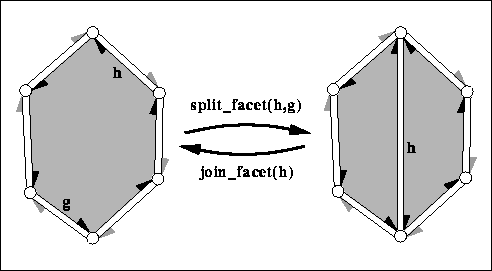## ◆ join_loop()

template<typename Traits >
 Halfedge_handle CGAL::Polyhedron_3< Traits >::join_loop ( Halfedge_handle h, Halfedge_handle g )

glues the boundary of the two facets denoted by h and g together and returns h.

Both facets and the vertices along the facet denoted by g gets removed. Both facets may be holes. The invariant join_loop( h, split_loop( h, i, j)) returns h and keeps the polyhedron unchanged.

Precondition
The facets denoted by h and g are different and have equal degree (i.e., number of edges).
Supports_removal must be CGAL::Tag_true.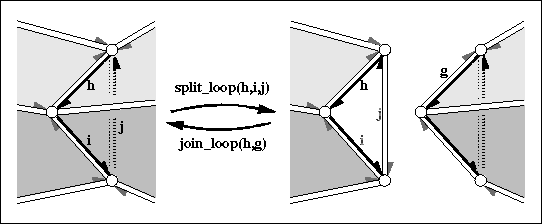## ◆ join_vertex()

template<typename Traits >
 Halfedge_handle CGAL::Polyhedron_3< Traits >::join_vertex ( Halfedge_handle h )

joins the two vertices incident to h.

The vertex denoted by h->opposite() gets removed. Returns the predecessor of h around the vertex, i.e., h->opposite()->prev(). The invariant join_vertex( split_vertex( h, g)) returns h and keeps the polyhedron unchanged. The time is proportional to the degree of the vertex removed and the time to compute h->prev() and h->opposite()->prev().

Precondition
The size of both facets incident to h is at least four (no multi-edges).
Supports_removal must be CGAL::Tag_true.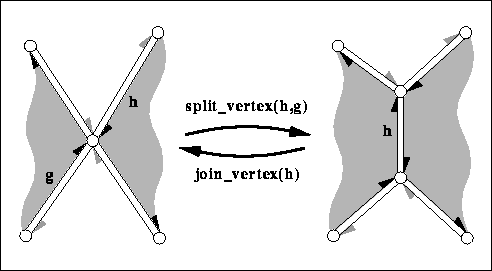## ◆ keep_largest_connected_components()

template<typename Traits >
 unsigned int CGAL::Polyhedron_3< Traits >::keep_largest_connected_components ( unsigned int nb_components_to_keep )

Erases the small connected components and the isolated vertices.

Keep nb_components_to_keep largest connected components. Returns the number of connected components erased (ignoring isolated vertices).

The polyhedron type must support vertices, halfedges, and removal operations.

Note that a stronger version of this function is offered by the package Polygon Mesh Processing : CGAL::Polygon_mesh_processing::keep_largest_connected_components() , which can be called since the class Polyhedron_3 is a model of the required concepts FaceListGraph and MutableFaceGraph (see The Class Polyhedron_3 as Model of the Boost Graph Concept for more information).

## ◆ make_hole()

template<typename Traits >
 Halfedge_handle CGAL::Polyhedron_3< Traits >::make_hole ( Halfedge_handle h )

removes the incident facet of h and changes all halfedges incident to the facet into border edges.

Returns h. See erase_facet(h) for a more generalized variant.

Precondition
None of the incident halfedges of the facet is a border edge.
Supports_removal must be CGAL::Tag_true.

## ◆ make_tetrahedron() [1/2]

template<typename Traits >
 Halfedge_handle CGAL::Polyhedron_3< Traits >::make_tetrahedron ( )

a tetrahedron is added to the polyhedral surface.

Returns a halfedge of the tetrahedron.

Examples:
Polyhedron/polyhedron_prog_color.cpp, and Polyhedron/polyhedron_prog_simple.cpp.

## ◆ make_tetrahedron() [2/2]

template<typename Traits >
 Halfedge_handle CGAL::Polyhedron_3< Traits >::make_tetrahedron ( const Point & p1, const Point & p2, const Point & p3, const Point & p4 )

a tetrahedron is added to the polyhedral surface with its vertices initialized to p1, p2, p3, and p4.

Returns that halfedge of the tetrahedron which incident vertex is initialized to p1. The incident vertex of the next halfedge is p2, and the vertex thereafter is p3. The remaining fourth vertex is initialized to p4.

## ◆ make_triangle() [1/2]

template<typename Traits >
 Halfedge_handle CGAL::Polyhedron_3< Traits >::make_triangle ( )

a triangle with border edges is added to the polyhedral surface.

Returns a non-border halfedge of the triangle.

## ◆ make_triangle() [2/2]

template<typename Traits >
 Halfedge_handle CGAL::Polyhedron_3< Traits >::make_triangle ( const Point & p1, const Point & p2, const Point & p3 )

a triangle with border edges is added to the polyhedral surface with its vertices initialized to p1, p2, and p3.

Returns that non-border halfedge of the triangle which incident vertex is initialized to p1. The incident vertex of the next halfedge is p2, and the vertex thereafter is p3.

## ◆ normalize_border()

template<typename Traits >
 void CGAL::Polyhedron_3< Traits >::normalize_border ( )

sorts halfedges such that the non-border edges precede the border edges.

For each border edge the halfedge iterator will reference the halfedge incident to the facet right before the halfedge incident to the hole.

## ◆ normalized_border_is_valid()

template<typename Traits >
 bool CGAL::Polyhedron_3< Traits >::normalized_border_is_valid ( bool verbose = false ) const

returns true if the border halfedges are in normalized representation, which is when enumerating all halfedges with the iterator: The non-border edges precede the border edges and for border edges, the second halfedge is the border halfedge.

The halfedge iterator border_halfedges_begin() denotes the first border edge. If verbose is true, statistics are printed to cerr.

## ◆ reserve()

template<typename Traits >
 void CGAL::Polyhedron_3< Traits >::reserve ( size_type v, size_type h, size_type f )

reserve storage for v vertices, h halfedges, and f facets.

The reservation sizes are a hint for optimizing storage allocation. If the capacity is already greater than the requested size nothing happens. If the capacity changes all iterators and circulators might invalidate.

## ◆ size_of_border_edges()

template<typename Traits >
 size_type CGAL::Polyhedron_3< Traits >::size_of_border_edges ( ) const

number of border edges.

Since each border edge of a polyhedral surface has exactly one border halfedge, this number is equal to size_of_border_halfedges().

Precondition
last normalize_border() call still valid, see above.

## ◆ size_of_border_halfedges()

template<typename Traits >
 size_type CGAL::Polyhedron_3< Traits >::size_of_border_halfedges ( ) const

number of border halfedges.

Precondition
last normalize_border() call still valid, see above.

## ◆ split_edge()

template<typename Traits >
 Halfedge_handle CGAL::Polyhedron_3< Traits >::split_edge ( Halfedge_handle h )

splits the halfedge h into two halfedges inserting a new vertex that is a copy of h->opposite()->vertex().

Is equivalent to split_vertex( h->prev(), h->opposite())->opposite(). The call of prev() can make this method slower than a direct call of split_vertex() if the previous halfedge is already known and computing it would be costly when the halfedge data structure does not support the prev() member function. Returns the new halfedge hnew pointing to the inserted vertex. The new halfedge is followed by the old halfedge, i.e., hnew->next() == h.

## ◆ split_facet()

template<typename Traits >
 Halfedge_handle CGAL::Polyhedron_3< Traits >::split_facet ( Halfedge_handle h, Halfedge_handle g )

splits the facet incident to h and g into two facets with a new diagonal between the two vertices denoted by h and g respectively.

The second (new) facet is a copy of the first facet. Returns h->next() after the operation, i.e., the new diagonal. The new face is to the right of the new diagonal, the old face is to the left. The time is proportional to the distance from h to g around the facet.

Precondition
h and g are incident to the same facet. h != g (no loops). h->next() != g and g->next() != h (no multi-edges).## ◆ split_loop()

template<typename Traits >
 Halfedge_handle CGAL::Polyhedron_3< Traits >::split_loop ( Halfedge_handle h, Halfedge_handle i, Halfedge_handle j )

cuts the polyhedron into two parts along the cycle (h,i,j) (edge j runs on the backside of the three dimensional figure above).

Three new vertices (one copy for each vertex in the cycle) and three new halfedges (one copy for each halfedge in the cycle), and two new triangles are created. h,i,j will be incident to the first new triangle. The return value will be the halfedge incident to the second new triangle which is the copy of h-opposite().

Precondition
h, i, j denote distinct, consecutive vertices of the polyhedron and form a cycle: i.e., h->vertex() == i->opposite()->vertex(), $$\ldots$$ , j->vertex() == h->opposite()->vertex(). The six facets incident to (h,i,j) are all distinct.## ◆ split_vertex()

template<typename Traits >
 Halfedge_handle CGAL::Polyhedron_3< Traits >::split_vertex ( Halfedge_handle h, Halfedge_handle g )

splits the vertex incident to h and g into two vertices, the old vertex remains and a new copy is created, and connects them with a new edge.

Let hnew be h->next()->opposite() after the split, i.e., a halfedge of the new edge. The split regroups the halfedges around the two vertices. The halfedge sequence hnew, g->next()->opposite(), $$\ldots$$ , h remains around the old vertex, while the halfedge sequence hnew->opposite(), h->next()->opposite() (before the split), $$\ldots$$ , g is regrouped around the new vertex. The split returns hnew, i.e., the new halfedge incident to the old vertex. The time is proportional to the distance from h to g around the vertex.

Precondition
h and g are incident to the same vertex. h != g (antennas are not allowed).Note
A special application of the split is split_vertex(h,h->next()->opposite()) which is equivalent to an edge split of the halfedge h->next() that creates a new vertex on the halfedge h->next(). See also split_edge(h) below.